# 价值与贝尔曼方程

## 值函数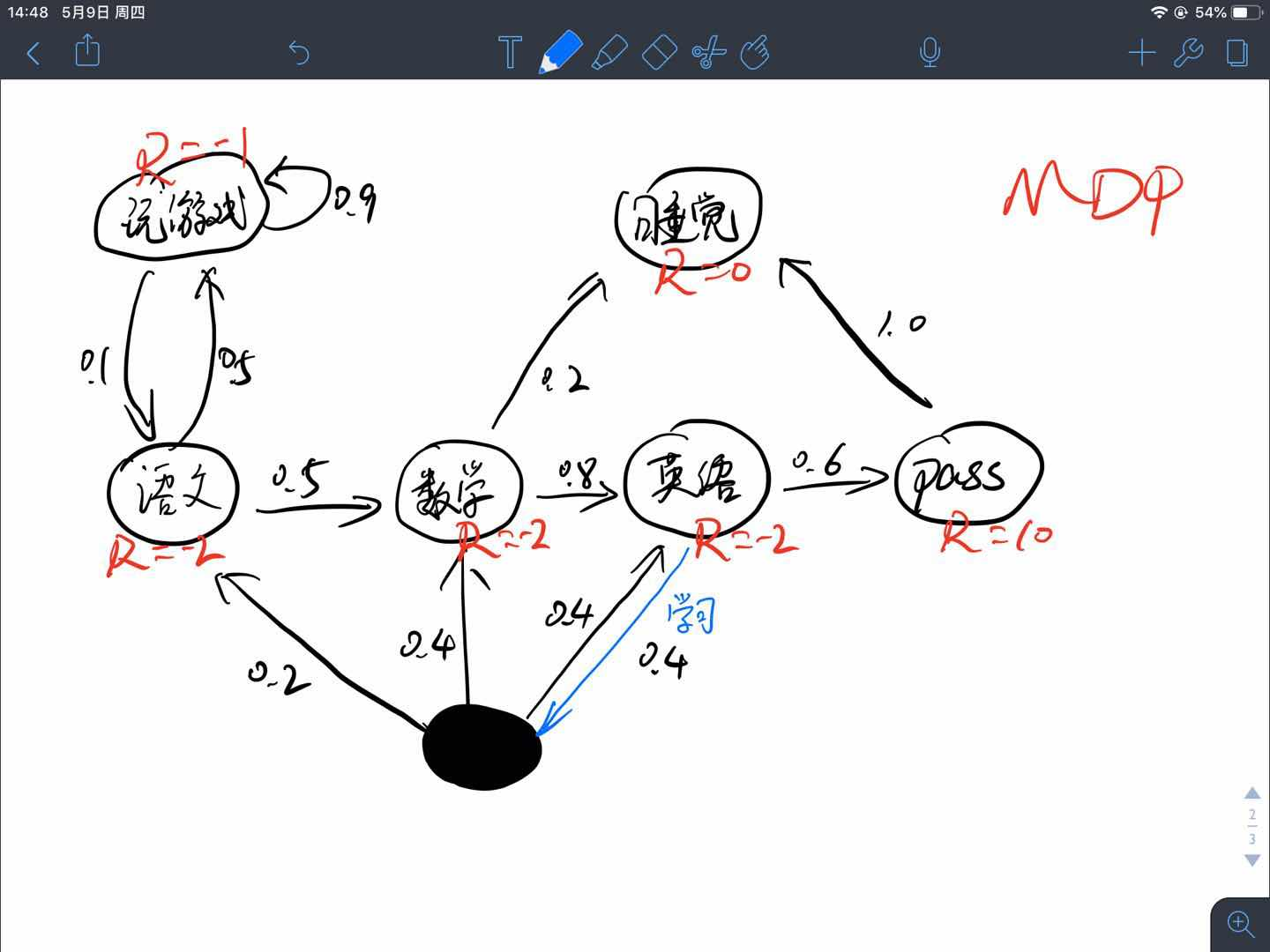A B C D E F
Reward -1 -2 -2 -2 10 0
A 0.9 0.1
B 0.5 0.5
C 0.8 0.2
D,0.4 0.2 0.4 0.4
D,0.6 0.6
E 1.0

### 状态值函数$V(s)$

$\pi$策略下$s$状态的价值函数可以表示为$v_{\pi}(s)$，由期望回报表示

## 贝尔曼方程

### 状态值函数$V(s)$与动作值函数$Q(s,a)$的关系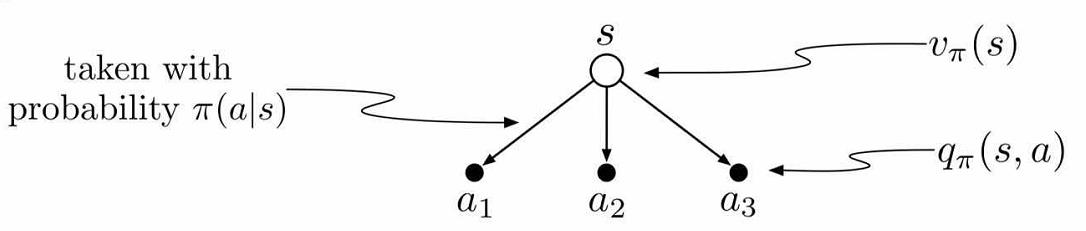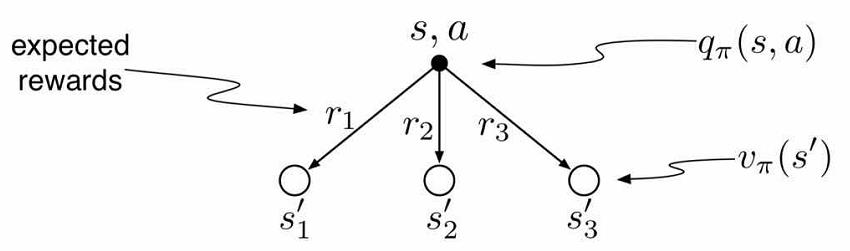### 贝尔曼期望方程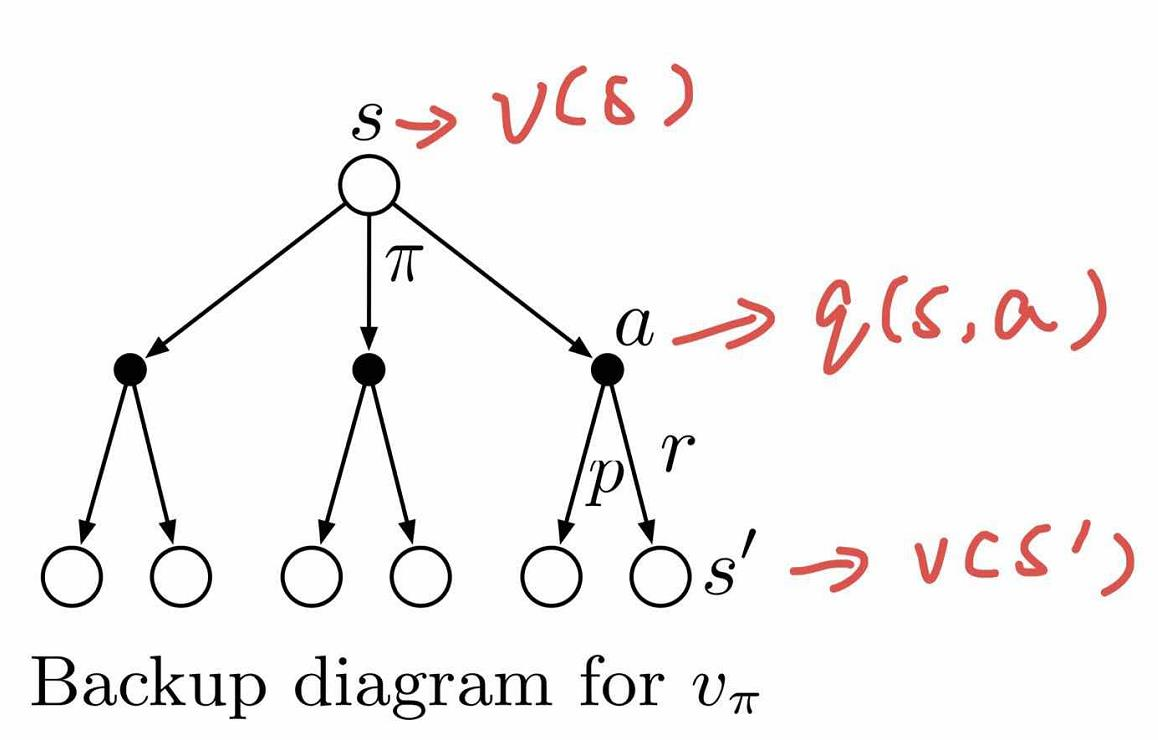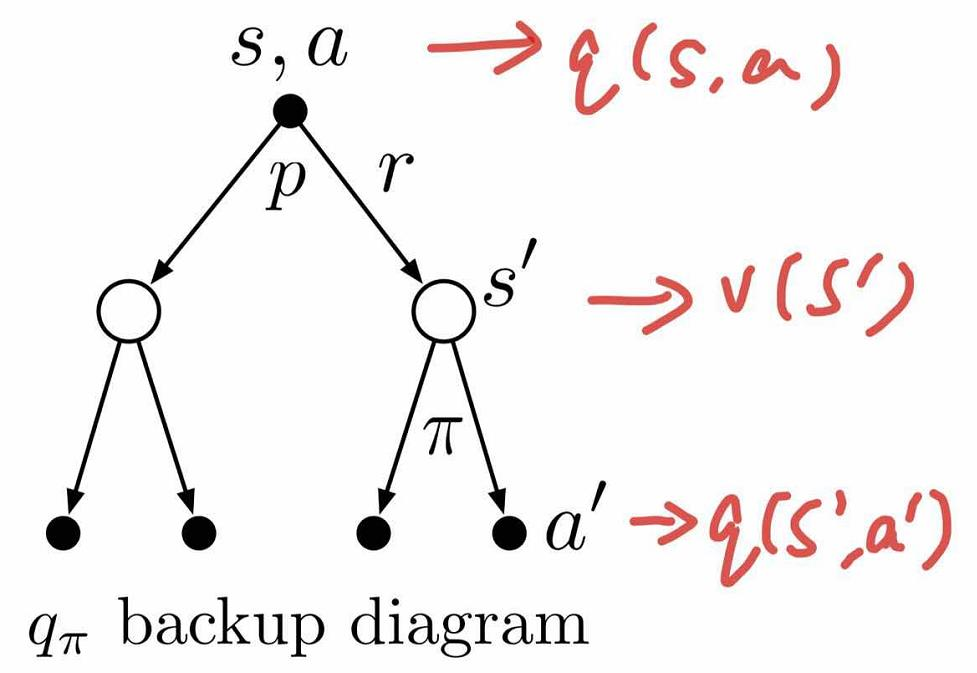### 贝尔曼最优方程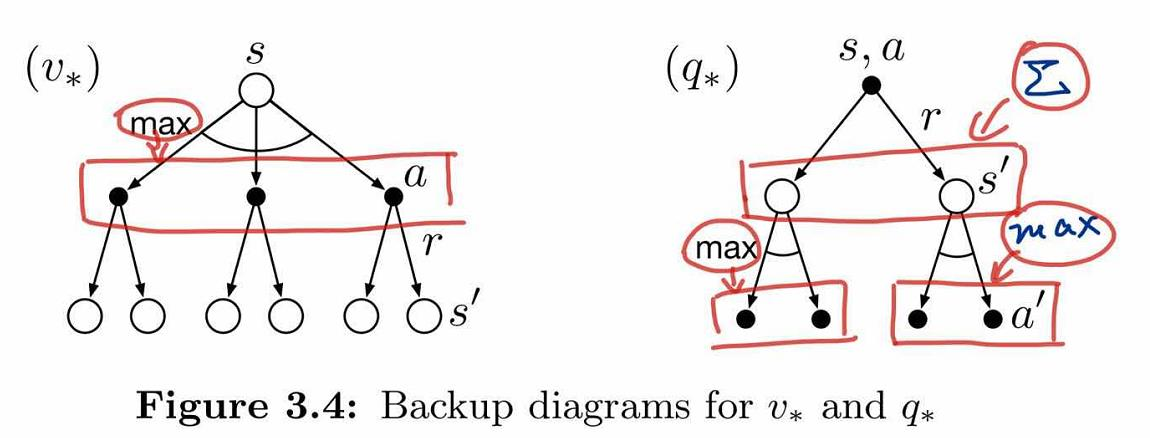### 最优策略

• 存在最优策略$\pi_{\ast}$，满足$\pi_{\ast} \geq \pi,\forall \pi$
• 所有最优策略的状态值函数都等于最优状态值函数$v_{\pi_{\ast}}(s)=v_{\ast}(s)$
• 所有最优策略的动作值函数都等于最优动作值函数$q_{\pi_{\ast}}(s,a)=q_{\ast}(s,a)$

## 例子

### 初始化全部状态值

V和Q，$\gamma =0.5$ 初始化V计算Q 迭代→V→Q 第46轮完全收敛
A 0 …… -2.171
B 0 …… -1.880
C 0 …… 0.651
D 0 …… 5.627
E 0 …… 0
F 0 …… 0
$(A,0.1)\rightarrow B$ 0 …… -2.940
$(A,0.9)\rightarrow A$ 0 …… -2.085
$(B,0.5_{A})\rightarrow A$ 0 …… -2.085
$(B,0.5_{C})\rightarrow C$ 0 …… -1.675
$(C,0.2)\rightarrow F$ 0 …… 0
$(C,0.8)\rightarrow D$ 0 …… 0.814
$(D,0.4)\rightarrow \begin{cases}B,0.2\\C,0.4\\D,0.4\end{cases}$ 0 …… -0.932
$(D,0.6)\rightarrow E$ 0 …… 10
$(E,1.0)\rightarrow F$ 0 …… 0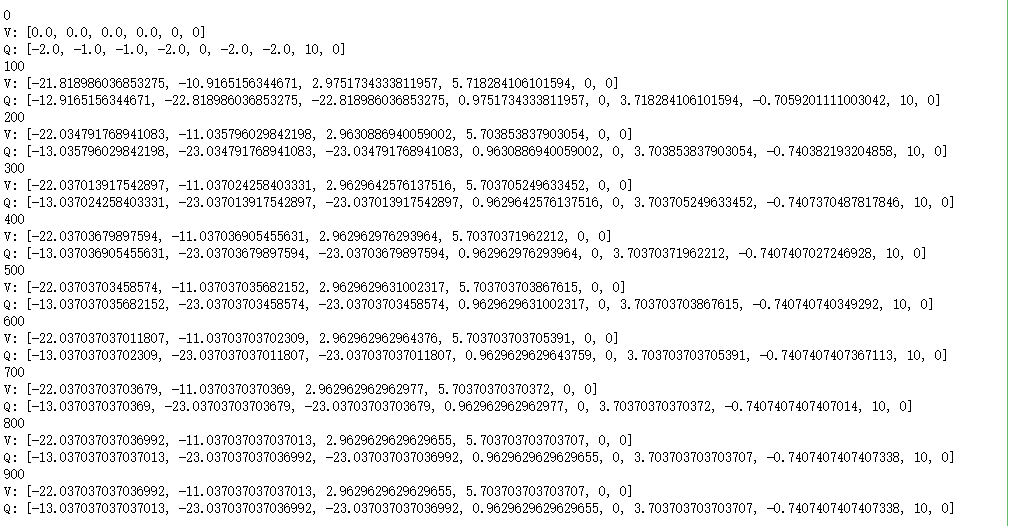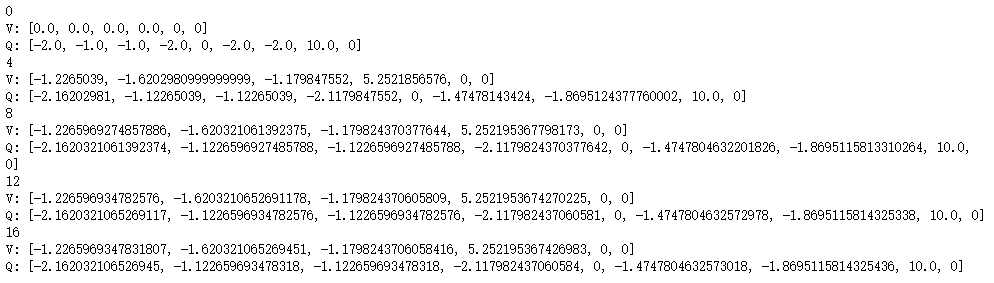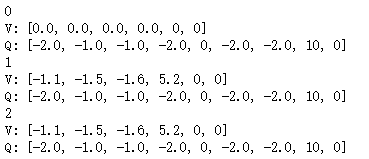• $\gamma =0$状态值最高的是$v(D)=5.2$，这很容易理解，D状态距离最大奖励值10最近，理应最好，这点与$\gamma =0.5$时相同。

• 但是对于状态C，$\gamma =0$时认为这个状态最差，$v(C)=-1.6$，$\gamma =0$时认为这个状态次优，$v(C)=0.651$，其实这就是目光短浅与目光长远的不同，$\gamma =0$并没有考虑到其附近状态的临近状态的价值，导致其主观的认为最接近我的都是负的，于是状态肯定差。

• 对于动作值也是一样，一个认为次优，一个认为最差。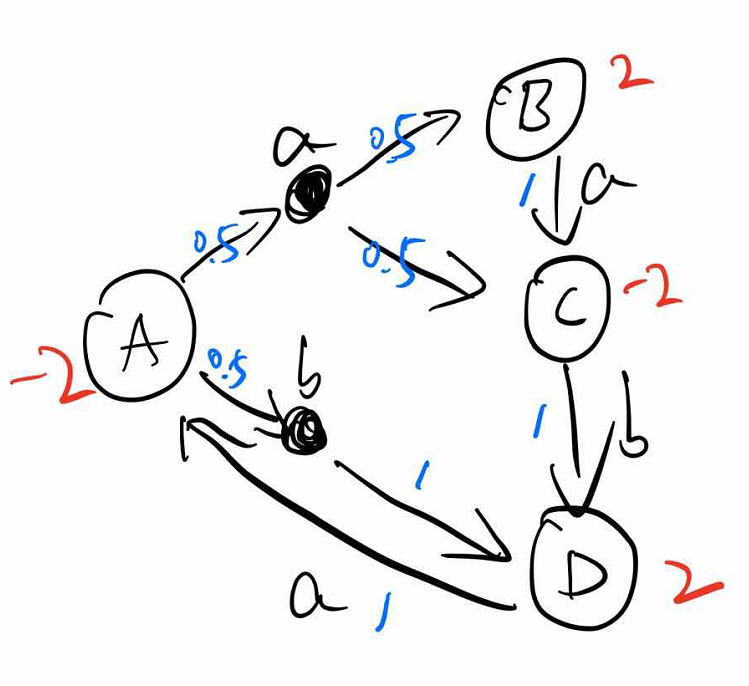$\gamma =1$时，迭代100W次也不收敛：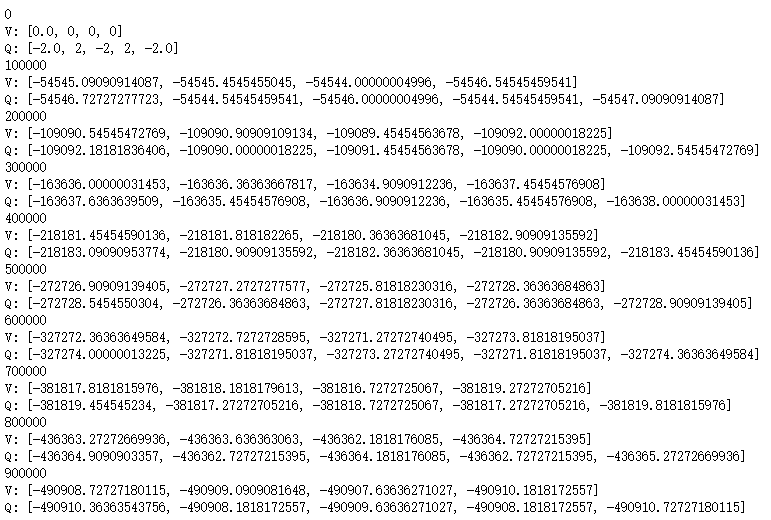$\gamma =0.5$时，迭代50多次即可收敛：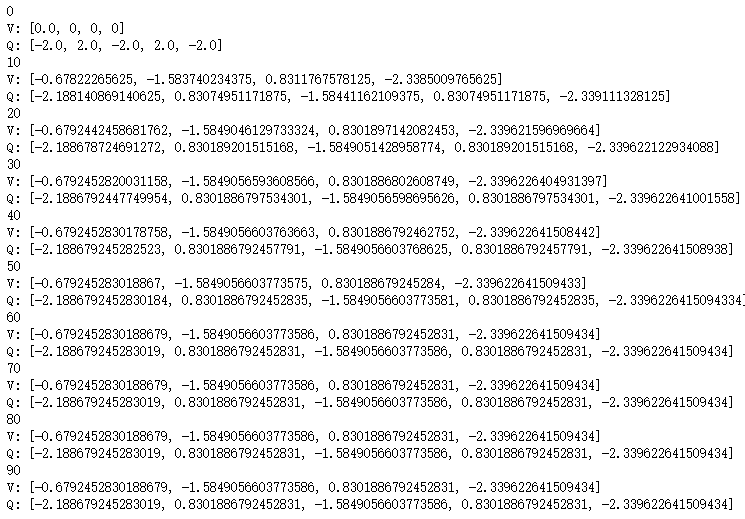-------------本文结束感谢您的阅读-------------

0%